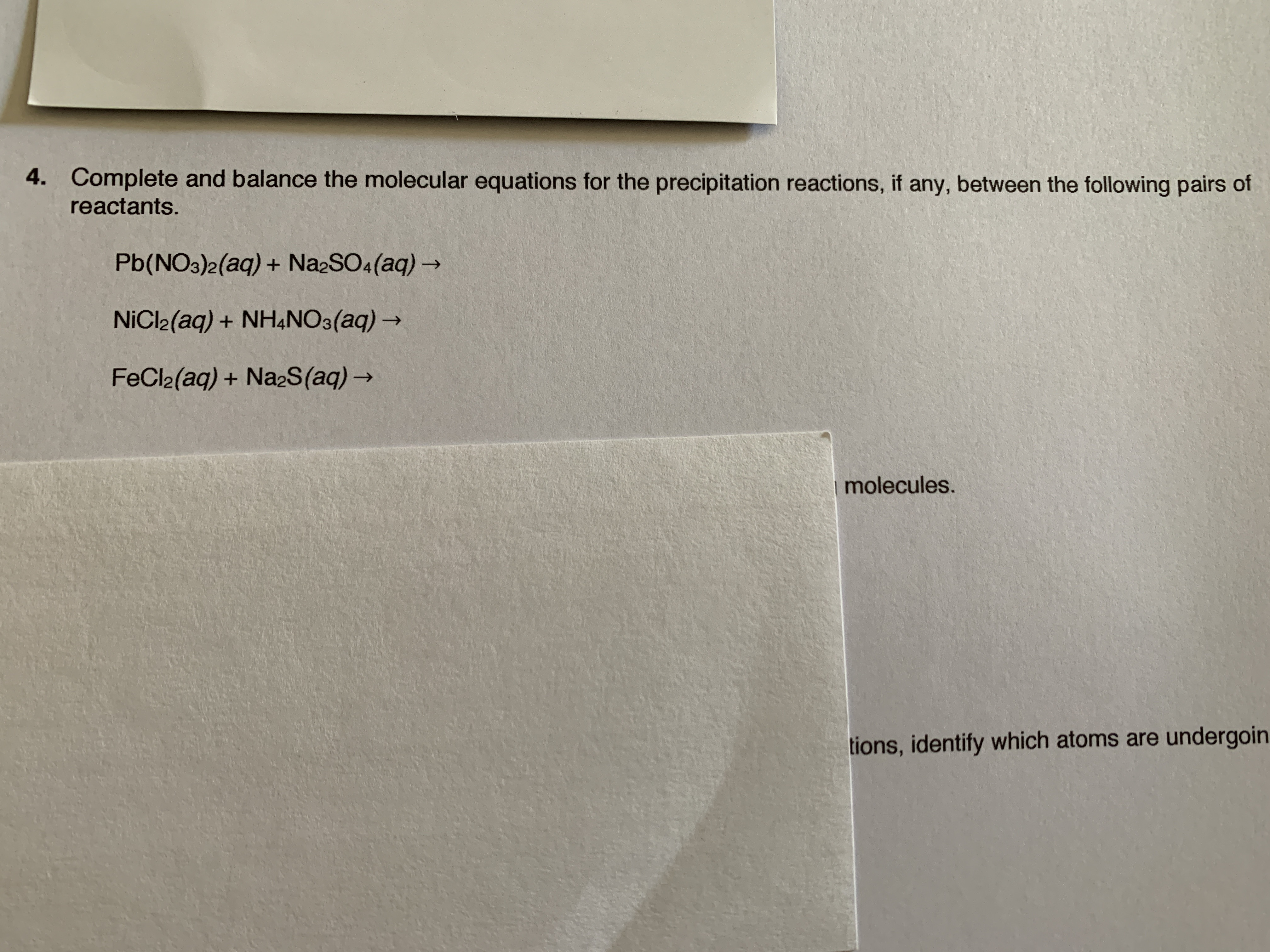# 4.Complete and balance the molecular equations for the precipitation reactions, if any, between the following pairs ofreactants.Pb(NO3)2(aq)+ Na2SO4(aq)NiCl2(aq) NH4NO3 (aq)FeCl2(aq) + Na2S (aq)molecules.tions, identify which atoms are undergoin

Question
83 viewshelp_outlineImage Transcriptionclose4. Complete and balance the molecular equations for the precipitation reactions, if any, between the following pairs of reactants. Pb(NO3)2(aq)+ Na2SO4(aq) NiCl2(aq) NH4NO3 (aq) FeCl2(aq) + Na2S (aq) molecules. tions, identify which atoms are undergoin fullscreen
check_circle

Step 1

Balanced chemical equation is defined as a chemical equation in which the product and reactant sides contain equal numbers of atoms of all the chemical species.

Step 2

The reaction of Pb(NO3)2 and Na2SO4 leads to the formation of the precipitate of PbSO4 and NaNO3 exists in the solution.

The chemical equation for the reaction of Pb(NO3)2 and Na2SO4 is labelled in the equation (1).

The balanced chemical equation for the reaction is represented in the equation (2).

Step 3

The reaction of NiCl2 and NH4NO3 does not form precipitate in the solution but it forms two products NiNO3 and NH4Cl in aqueous form.

The chemical equation for the reaction of NiCl2 and NH4NO3...

### Want to see the full answer?

See Solution

#### Want to see this answer and more?

Solutions are written by subject experts who are available 24/7. Questions are typically answered within 1 hour.*

See Solution
*Response times may vary by subject and question.
Tagged in